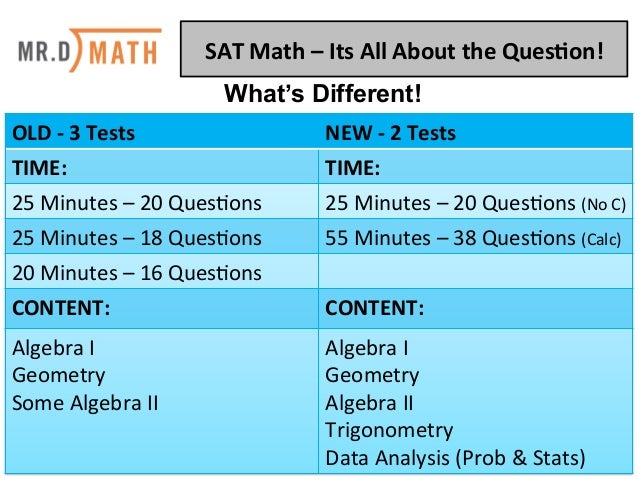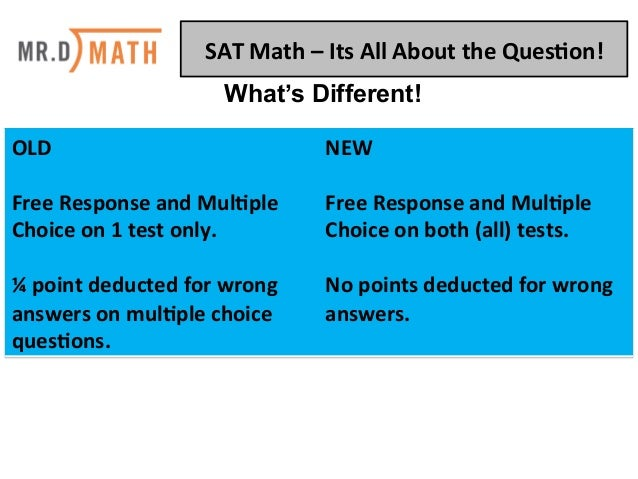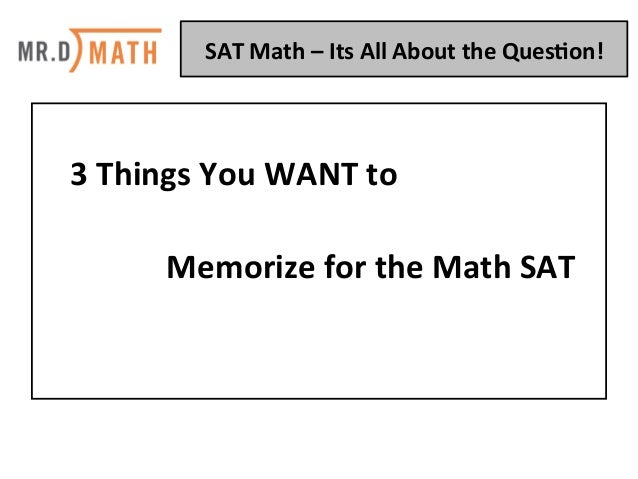Successfully reported this slideshow.You’ve finished this document.
Upcoming SlideShare
Next

ofUpcoming SlideShare
Next

Share

# SAT Math: It's All About The Question

Join Mr. D for a mini-SAT Boot Camp. Mr. D will be showing the tips and techniques from his SAT Boot Camps. This workshop will focus on math, the language behind the questions and show participants what they really need to know before taking the math section of the SAT.
Find out the words on the test that give tips for how to solve the questions as well learning what formulas you really need to know before the taking the SAT. Students and parents alike will learn how to unravel the questions being asked into something they can solve quickly and easily. These techniques can be used for other testing situations and subject areas as well.

See all

### Related Audiobooks

#### Free with a 30 day trial from Scribd

See all
• Be the first to like this

### SAT Math: It's All About The Question

1. 1. SAT  Math  –  Its  All  About  the  Ques2on!   OLD  -­‐  3  Tests   NEW  -­‐  2  Tests   TIME:   TIME:   25  Minutes  –  20  Ques.ons   25  Minutes  –  20  Ques.ons  (No  C)     25  Minutes  –  18  Ques.ons   55  Minutes  –  38  Ques.ons  (Calc)   20  Minutes  –  16  Ques.ons   CONTENT:   CONTENT:   Algebra  I   Geometry   Some  Algebra  II   Algebra  I   Geometry   Algebra  II   Trigonometry   Data  Analysis  (Prob  &  Stats)   What’s Different!
2. 2. SAT  Math  –  Its  All  About  the  Ques2on!   OLD     Free  Response  and  Mul2ple   Choice  on  1  test  only.     ¼  point  deducted  for  wrong   answers  on  mul2ple  choice   ques2ons.   NEW     Free  Response  and  Mul2ple   Choice  on  both  (all)  tests.     No  points  deducted  for  wrong   answers.     What’s Different!
3. 3.    3  Things  You  WANT  to                  Memorize  for  the  Math  SAT       SAT  Math  –  Its  All  About  the  Ques2on!
4. 4. 1.  The  Pythagorean  Theorem    a2  +  b2  =  c2     •  The  Pythagorean  Theorem  is  used  for   ﬁnding  the  length  of  the  hypotenuse  of  a   right  triangle.  While  that  is  great  and   useful,  it  can  be  used  for  much  more.       SAT  Math  –  Its  All  About  the  Ques2on!   a
5. 5. The  Pythagorean  Theorem    a2  +  b2  =  c2     •  Instead  of  memorizing  the  distance  formula,   you  can  simply  use  the  Pythagorean  Theorem.     •  The  30-­‐60-­‐90  and  45-­‐45-­‐90  special  right   triangle  formulas  are  given  on  the  SAT,  the   Pythagorean  Theorem  can  be  used  in  place  of   those  too.     SAT  Math  –  Its  All  About  the  Ques2on!   a
6. 6. 2.  Pythagorean  Triples   •  Here  are  the  two  Pythagorean  Triples   that  will  show  up  most  oWen  on  the  SAT:                          3-­‐4-­‐5        as  32  +  42  =  52                          5-­‐12-­‐13  as  52  +  122  =  132       SAT  Math  –  Its  All  About  the  Ques2on!   a
7. 7. You  can  use  those  same  Pythagorean  Triples  to   make  more  of  them.  What  do  I  mean  by  “make   more  of  them?”  Just  count  by  each  number  to   make  more.  For  example,  count  by  3’s,  4’s  and   5’s!                  3-­‐4-­‐5  will  also  create  as  6-­‐8-­‐10,  and                9-­‐12-­‐15,  and  so  on.                  5-­‐12-­‐13  will  do  the  same  as  10-­‐24-­‐26.     SAT  Math  –  Its  All  About  the  Ques2on!   a
8. 8. 3.  Know  what  f(x)  means   •  f(x)  is  called  func.on  nota.on  and  is  read   f  of  x.   •  f(x)  is  just  a  fancy  way  to  say  “y”  in  an   equa.on  that  also  has  an  “x.”          f(x)  =  2x  +  5  really  means  y  =  2x  +  5   SAT  Math  –  Its  All  About  the  Ques2on!   a
9. 9. 3.  Know  what  f(x)  means   •  f(3)  is  read  f  at  3.        If  f(x)  =  2x  +  5  ﬁnd  f(3).      Replace  x  with  3!          f(3)  =  2(3)  +  5      f(3)  =  6  +  5      f(3)  =  11   SAT  Math  –  Its  All  About  the  Ques2on!   a
10. 10. 3.  Know  what  f(x)  means   •  Find  x  if  f(x)  =  3  when  f(x)  =  2x  +  5.              Replace  f(x)  with  3!          3  =  2x  +  5        -­‐2  =  2x        -­‐1  =  x   SAT  Math  –  Its  All  About  the  Ques2on!   a
11. 11. Mr.  D’s  2  Step  Method  for  Taking  Tests            (any  test/any  subject)       Step  1  -­‐  Find  the  Ques.on   Step  2  -­‐  Answer  the  Ques.on     SAT  Math  –  Its  All  About  the  Ques2on!
12. 12. SAT  Math  –  Its  All  About  the  Ques2on!   On Saturday afternoon, Armand sent m text messages each hour for 5 hours, and Tyrone sent p text messages each hour for 4 hours. Which of the following represents the total number of messages sent by Armand and Tyrone on Saturday afternoon? A) 9mp B) 20mp C) 5m + 4p D) 4m + 5p
13. 13. SAT  Math  –  Its  All  About  the  Ques2on!   On Saturday afternoon, Armand sent m text messages each hour for 5 hours, and Tyrone sent p text messages each hour for 4 hours. Which of the following represents the total number of messages sent by Armand and Tyrone on Saturday afternoon? A) 9mp B) 20mp C) 5m + 4p D) 4m + 5p
14. 14. SAT  Math  –  Its  All  About  the  Ques2on!   Kathy is a repair technician for a phone company. Each week, she receives a batch of phones that need repairs. The number of phones that she has left to fix at the end of each day can be estimated with the equation P = 108 − 23d, where P is the number of phones left and d is the number of days she has worked that week. What is the meaning of the value 108 in this equation? A) Kathy will complete the repairs within 108 days. B) Kathy starts each week with 108 phones to fix. C) Kathy repairs phones at a rate of 108 per hour. D) Kathy repairs phones at a rate of 108 per day.
15. 15. SAT  Math  –  Its  All  About  the  Ques2on!   Kathy is a repair technician for a phone company. Each week, she receives a batch of phones that need repairs. The number of phones that she has left to fix at the end of each day can be estimated with the equation P = 108 − 23d, where P is the number of phones left and d is the number of days she has worked that week. What is the meaning of the value 108 in this equation? A y-intercept is when x = 0 or when time begins! A) Kathy will complete the repairs within 108 days. B) Kathy starts each week with 108 phones to fix. C) Kathy repairs phones at a rate of 108 per hour. D) Kathy repairs phones at a rate of 108 per day.
16. 16. SAT  Math  –  Its  All  About  the  Ques2on!
17. 17. SAT  Math  –  Its  All  About  the  Ques2on!
18. 18. SAT  Math  –  Its  All  About  the  Ques2on!
19. 19. SAT  Math  –  Its  All  About  the  Ques2on!
20. 20. SAT  Math  –  Its  All  About  the  Ques2on!   A function f satisfies f(2) = 3 and f(3) = 5. A function g satisfies g(3) = 2 and g(5) = 6. What is the value of f(g(3))? A) 2 B) 3 C) 5 D) 6 Since g(3) = 2, f(g(3)) = f(2) f(2) = 3
21. 21. SAT  Math  –  Its  All  About  the  Ques2on!   A function f satisfies f(2) = 3 and f(3) = 5. A function g satisfies g(3) = 2 and g(5) = 6. What is the value of f(g(3))? A) 2 B) 3 C) 5 D) 6 Since g(3) = 2, f(g(3)) = f(2) f(2) = 3
22. 22. SAT  Math  –  Its  All  About  the  Ques2on!   If 16 + 4x is 10 more than 14, what is the value of 8x? A) 2 B) 6 C) 16 D) 80
23. 23. SAT  Math  –  Its  All  About  the  Ques2on!   If 16 + 4x is 10 more than 14, what is the value of 8x? A) 2 B) 6 C) 16 D) 80 16 + 4x = 24 Multiply by 2 to get 8x 32 + 8x = 48 8x = 16
24. 24. SAT  Math  –  Its  All  About  the  Ques2on!   The complete graph of the function f is shown in the xy-plane above. For what value of x is the value of f(x) at its minimum? A) −5 C) −2 B) −3 D) 3
25. 25. SAT  Math  –  Its  All  About  the  Ques2on!   The complete graph of the function f is shown in the xy-plane above. For what value of x is the value of f(x) at its minimum? A) −5 C) −2 B) −3 D) 3
26. 26. SAT  Math  –  Its  All  About  the  Ques2on!   The data in the table above were produced by a sleep researcher studying the number of dreams people recall when asked to record their dreams for one week. Group X consisted of 100 people who observed early bedtimes, and Group Y consisted of 100 people who observed later bedtimes. If a person is chosen at random from those who recalled at least 1 dream, what is the probability that the person belonged to Group Y ? A) 68 C) 79 100 164 B) 79 D) 164 100 200
27. 27. SAT  Math  –  Its  All  About  the  Ques2on!   The data in the table above were produced by a sleep researcher studying the number of dreams people recall when asked to record their dreams for one week. Group X consisted of 100 people who observed early bedtimes, and Group Y consisted of 100 people who observed later bedtimes. If a person is chosen at random from those who recalled at least 1 dream, what is the probability that the person belonged to Group Y ? A) 68 C) 79 100 164 B) 79 D) 164 100 200
28. 28. SAT  Math  –  Its  All  About  the  Ques2on!   A researcher conducted a survey to determine whether people in a certain large town prefer watching sports on television to attending the sporting event. The researcher asked 117 people who visited a local restaurant on a Saturday, and 7 people refused to respond. Which of the following factors makes it least likely that a reliable conclusion can be drawn about the sports-watching preferences of all people in the town? A) Sample size B) Population size C) The number of people who refused to respond D) Where the survey was given
29. 29. SAT  Math  –  Its  All  About  the  Ques2on!   A researcher conducted a survey to determine whether people in a certain large town prefer watching sports on television to attending the sporting event. The researcher asked 117 people who visited a local restaurant on a Saturday, and 7 people refused to respond. Which of the following factors makes it least likely that a reliable conclusion can be drawn about the sports-watching preferences of all people in the town? A) Sample size B) Population size C) The number of people who refused to respond D) Where the survey was given Statistics is all about surveys being done randomly!

Total views

19,647

On Slideshare

0

From embeds

0

Number of embeds

19,086

7

Shares

0